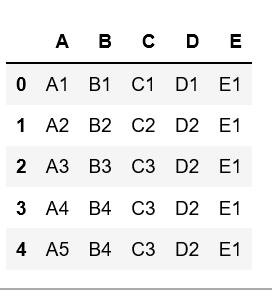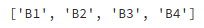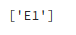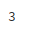Related Articles
Get unique values from a column in Pandas DataFrame
• Last Updated : 10 Dec, 2018

Let’s discuss how to get unique values from a column in Pandas DataFrame.

Create a simple dataframe with dictionary of lists, say columns name are A, B, C, D, E with duplicate elements.Now, let’s get the unique values of a column in this dataframe.

Example #1: Get the unique values of ‘B’ column

 `# Import pandas package ``import` `pandas as pd`` ` `# create a dictionary with five fields each``data ``=` `{``    ``'A'``:[``'A1'``, ``'A2'``, ``'A3'``, ``'A4'``, ``'A5'``], ``    ``'B'``:[``'B1'``, ``'B2'``, ``'B3'``, ``'B4'``, ``'B4'``], ``    ``'C'``:[``'C1'``, ``'C2'``, ``'C3'``, ``'C3'``, ``'C3'``], ``    ``'D'``:[``'D1'``, ``'D2'``, ``'D2'``, ``'D2'``, ``'D2'``], ``    ``'E'``:[``'E1'``, ``'E1'``, ``'E1'``, ``'E1'``, ``'E1'``] }`` ` `# Convert the dictionary into DataFrame ``df ``=` `pd.DataFrame(data)`` ` `# Get the unique values of 'B' column``df.B.unique()`

Output:Example #2: Get the unique values of ‘E’ column

 `# Import pandas package ``import` `pandas as pd`` ` `# create a dictionary with five fields each``data ``=` `{``    ``'A'``:[``'A1'``, ``'A2'``, ``'A3'``, ``'A4'``, ``'A5'``], ``    ``'B'``:[``'B1'``, ``'B2'``, ``'B3'``, ``'B4'``, ``'B4'``], ``    ``'C'``:[``'C1'``, ``'C2'``, ``'C3'``, ``'C3'``, ``'C3'``], ``    ``'D'``:[``'D1'``, ``'D2'``, ``'D2'``, ``'D2'``, ``'D2'``], ``    ``'E'``:[``'E1'``, ``'E1'``, ``'E1'``, ``'E1'``, ``'E1'``] }`` ` `# Convert the dictionary into DataFrame ``df ``=` `pd.DataFrame(data)`` ` `# Get the unique values of 'E' column``df.E.unique()`

Output:Example #3: Get number of unique values in a column

 `# Import pandas package ``import` `pandas as pd`` ` `# create a dictionary with five fields each``data ``=` `{``    ``'A'``:[``'A1'``, ``'A2'``, ``'A3'``, ``'A4'``, ``'A5'``], ``    ``'B'``:[``'B1'``, ``'B2'``, ``'B3'``, ``'B4'``, ``'B4'``], ``    ``'C'``:[``'C1'``, ``'C2'``, ``'C3'``, ``'C3'``, ``'C3'``], ``    ``'D'``:[``'D1'``, ``'D2'``, ``'D2'``, ``'D2'``, ``'D2'``], ``    ``'E'``:[``'E1'``, ``'E1'``, ``'E1'``, ``'E1'``, ``'E1'``] }`` ` `# Convert the dictionary into DataFrame ``df ``=` `pd.DataFrame(data)`` ` `# Get number of unique values in column 'C'``df.C.nunique(dropna ``=` `True``)`

Output:Attention geek! Strengthen your foundations with the Python Programming Foundation Course and learn the basics.

To begin with, your interview preparations Enhance your Data Structures concepts with the Python DS Course. And to begin with your Machine Learning Journey, join the Machine Learning – Basic Level Course

My Personal Notes arrow_drop_up